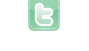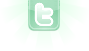## how do you make an object point away from another object?

im trying to make a game where an ant is chasing lots of other small and im trying to make them face away and i don't know how any ideas?
You could take a look at the source code of my 'Catch the fly scenario' ( http://greenfootgallery.org/scenarios/3221 )
This is the code you might be interested in. Write this in your chased Actor:
```Ant a = new Ant();
int x = a.getX() - getX();
int y = a.getY() - getY();
r = Math.toDegrees(Math.atan2(x, y)) - 270;
setRotation(-(int) r);
move(...speed...);```
my editor says " cannot find symbol - variable r " what do i do?
Oh sorry forgot an 'int' :
```Ant a = new Ant();
int x = a.getX() - getX();
int y = a.getY() - getY();
[b]int[/b] r = Math.toDegrees(Math.atan2(x, y)) - 270;
setRotation(-(int) r);
move(...speed...);  ```
Oh sorry forgot an 'int' : view plaincopy to clipboardprint? Ant a = new Ant(); int x = a.getX() - getX(); int y = a.getY() - getY(); int r = Math.toDegrees(Math.atan2(x, y)) - 270; setRotation(-(int) r); move(...speed...);
my editor looks like this: Ant a = new Ant(); int x = a.getX() - getX(); int y = a.getY() - getY(); int r = Math.toDegrees(Math.atan2(x, y)) - 270; <- It says here " possible loss of precision found setRotation(-(int) r); :doublerquired: int " move(2);
Upps, r has to be a double. so its double r = Math..........
soory again but this terminal error thingy keeps popping up when i press the run button and saying java.lang.IllegalStateException: The actor has not been inserted into a world so it has no location yet. You might want to look at the method addedToWorld on the Actor class. at greenfoot.Actor.failIfNotInWorld(Actor.java:659) at greenfoot.Actor.getX(Actor.java:157) at EvenSmallerAnt.turnAway(EvenSmallerAnt.java:51) at EvenSmallerAnt.act(EvenSmallerAnt.java:18) at greenfoot.core.Simulation.actActor(Simulation.java:498) at greenfoot.core.Simulation.runOneLoop(Simulation.java:461) at greenfoot.core.Simulation.runContent(Simulation.java:197) at greenfoot.core.Simulation.run(Simulation.java:187)
You cannot call getX() or getY() from the constructor of an object (public ()) Move the code that uses these calls into the act method and you should be good.